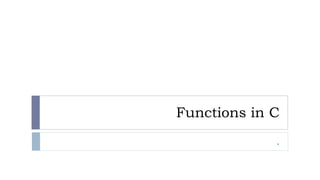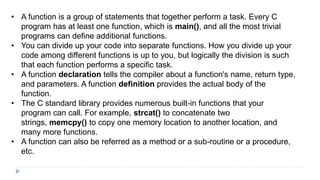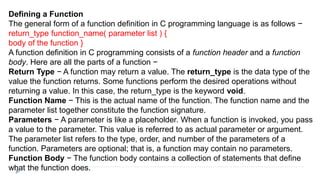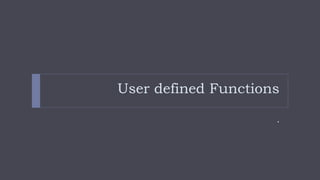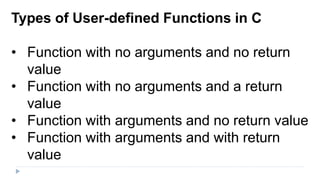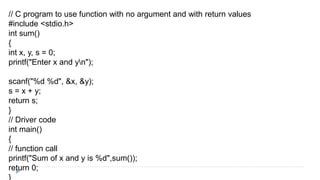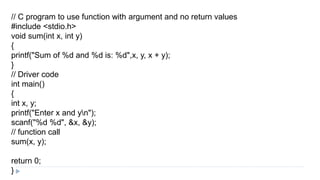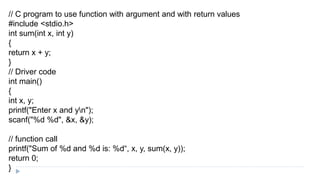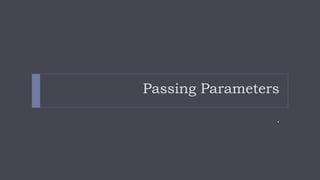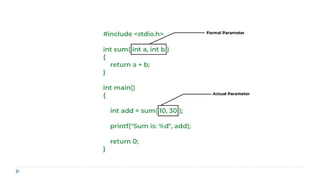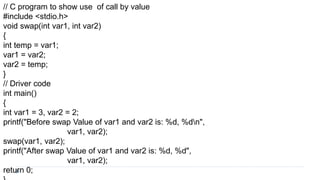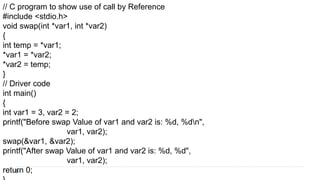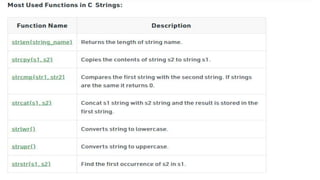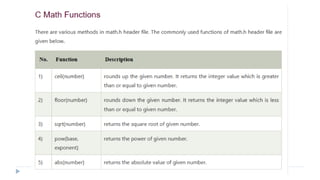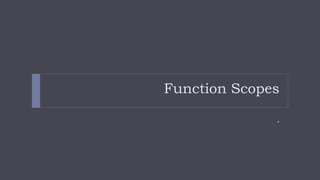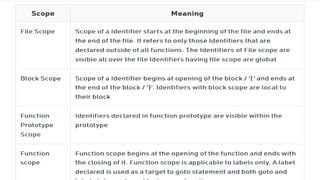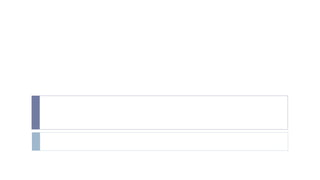1 of 19

### Functions in C.pptx

1. Functions in C .
2. • A function is a group of statements that together perform a task. Every C program has at least one function, which is main(), and all the most trivial programs can define additional functions. • You can divide up your code into separate functions. How you divide up your code among different functions is up to you, but logically the division is such that each function performs a specific task. • A function declaration tells the compiler about a function's name, return type, and parameters. A function definition provides the actual body of the function. • The C standard library provides numerous built-in functions that your program can call. For example, strcat() to concatenate two strings, memcpy() to copy one memory location to another location, and many more functions. • A function can also be referred as a method or a sub-routine or a procedure, etc.
3. Defining a Function The general form of a function definition in C programming language is as follows − return_type function_name( parameter list ) { body of the function } A function definition in C programming consists of a function header and a function body. Here are all the parts of a function − Return Type − A function may return a value. The return_type is the data type of the value the function returns. Some functions perform the desired operations without returning a value. In this case, the return_type is the keyword void. Function Name − This is the actual name of the function. The function name and the parameter list together constitute the function signature. Parameters − A parameter is like a placeholder. When a function is invoked, you pass a value to the parameter. This value is referred to as actual parameter or argument. The parameter list refers to the type, order, and number of the parameters of a function. Parameters are optional; that is, a function may contain no parameters. Function Body − The function body contains a collection of statements that define what the function does.
4. User defined Functions .
5. Types of User-defined Functions in C • Function with no arguments and no return value • Function with no arguments and a return value • Function with arguments and no return value • Function with arguments and with return value
6. // C program to use function with no argument and no return values #include <stdio.h> void sum() { int x, y; printf("Enter x and yn"); scanf("%d %d", &x, &y); printf("Sum of %d and %d is: %d", x, y, x + y); } // Driver code int main() { // function call sum(); return 0; }
7. // C program to use function with no argument and with return values #include <stdio.h> int sum() { int x, y, s = 0; printf("Enter x and yn"); scanf("%d %d", &x, &y); s = x + y; return s; } // Driver code int main() { // function call printf("Sum of x and y is %d",sum()); return 0; }
8. // C program to use function with argument and no return values #include <stdio.h> void sum(int x, int y) { printf("Sum of %d and %d is: %d",x, y, x + y); } // Driver code int main() { int x, y; printf("Enter x and yn"); scanf("%d %d", &x, &y); // function call sum(x, y); return 0; }
9. // C program to use function with argument and with return values #include <stdio.h> int sum(int x, int y) { return x + y; } // Driver code int main() { int x, y; printf("Enter x and yn"); scanf("%d %d", &x, &y); // function call printf("Sum of %d and %d is: %d“, x, y, sum(x, y)); return 0; }
10. Passing Parameters .
11. // C program to show use of call by value #include <stdio.h> void swap(int var1, int var2) { int temp = var1; var1 = var2; var2 = temp; } // Driver code int main() { int var1 = 3, var2 = 2; printf("Before swap Value of var1 and var2 is: %d, %dn", var1, var2); swap(var1, var2); printf("After swap Value of var1 and var2 is: %d, %d", var1, var2); return 0;
12. // C program to show use of call by Reference #include <stdio.h> void swap(int *var1, int *var2) { int temp = *var1; *var1 = *var2; *var2 = temp; } // Driver code int main() { int var1 = 3, var2 = 2; printf("Before swap Value of var1 and var2 is: %d, %dn", var1, var2); swap(&var1, &var2); printf("After swap Value of var1 and var2 is: %d, %d", var1, var2); return 0;
13. Function Scopes .